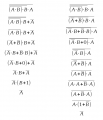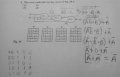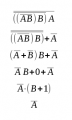# Boolean equation help

#### electronicsbeginner12

Joined Dec 14, 2014
27
Hi. Im having a problem solving the boolean equation in the attached file. I have worked out the problem, but am still getting the wrong answer for output C. Did I set up the equation correctly? Thanks in advance.

#### shteii01

Joined Feb 19, 2010
4,644
Um... why setup equation at all?
Just plug each pair of inputs A and B, and get the C. You got 4 sets of inputs A and B, get the 4 C, then compare your truth table to the provided 4 and mark which of the 4 matches the one you made. Should take about 20 minutes.

#### electronicsbeginner12

Joined Dec 14, 2014
27
Um... why setup equation at all?
Just plug each pair of inputs A and B, and get the C. You got 4 sets of inputs A and B, get the 4 C, then compare your truth table to the provided 4 and mark which of the 4 matches the one you made. Should take about 20 minutes.
Yes. Its possible to do it the way you describe, but the problem in my text is asking for the output equation.

There seems to be a mismatch here....
View attachment 95377
Thanks for pointing that out. I have reworked the problem and have arrived at the right answer. Can someone please verify if my steps are logical. Thanks.

#### djsfantasi

Joined Apr 11, 2010
8,884
You've made a mistake in your second step, while applying DeMorgans. However, you end with the correct answer in the third step with a second error. I would take a few more steps to split the terms. Similarly, in the third to last step, you have another error, but once again serendipitously arrive at the correct answer. Hint: (ab)'*b is NOT equal to a'+b'*b...

#### electronicsbeginner12

Joined Dec 14, 2014
27
You've made a mistake in your second step, while applying DeMorgans. However, you end with the correct answer in the third step with a second error. I would take a few more steps to split the terms. Similarly, in the third to last step, you have another error, but once again serendipitously arrive at the correct answer. Hint: (ab)'*b is NOT equal to a'+b'*b...
I tried putting a parenthesis to make it more clear. In the second step, i broke the double negation above the * sign and kept the sign because its a double negation. When i applied DeMorgans theorem above ab', it becomes a'+b' in the 4th step. B'*B becomes zero in the 5th step and a'+a' equals a' in the last step. I am confused as to how Im arriving at the right answer if the steps are wrong. Ive attached the rework of the problem.

#### RBR1317

Joined Nov 13, 2010
706
I tried putting a parenthesis to make it more clear. In the second step, i broke the double negation...
In order to improve clarity, never break a double negation. A double negation cancels itself; just eliminate it. One rule I follow is to only break one overbar at a time. I believe the attached image provides a sufficient level of clarity. Note the two methods involved: one method breaks overbars from the outside in, the other from the inside out.#### electronicsbeginner12

Joined Dec 14, 2014
27
In order to improve clarity, never break a double negation. A double negation cancels itself; just eliminate it. One rule I follow is to only break one overbar at a time. I believe the attached image provides a sufficient level of clarity. Note the two methods involved: one method breaks overbars from the outside in, the other from the inside out.
I reworked the problem using the outside in method you showed and have attached the image. Did I take logical steps in solving the problem?

From what you showed, it looks like there are less steps involved with the outside in method compared to the inside out method. Is this correct? Thanks.Mod note: reduced image file size

Last edited by a moderator:

#### djsfantasi

Joined Apr 11, 2010
8,884
Last edited by a moderator:

#### RBR1317

Joined Nov 13, 2010
706
•djsfantasi

#### electronicsbeginner12

Joined Dec 14, 2014
27
View attachment 95475 How did you get the write answer if you made a mistake? Dumb luck. Remember my hint? (ab)'*b is not equal to a'+b'*b. You have to distribute the b term over the entire expanded (ab)'. See above. Note this starts at step 3 in RBR1317's solution.
Thanks for working the problem out. I see now where I made the mistake.

It is not correct if you skip necessary steps. Note in the attached image that there are implied parentheses that must become real when you break the overbar. Why is that?
View attachment 95493
Is that because when i break the overbar, it becomes two terms with the same operation? The B that has a different operation is distributed in?

#### WBahn

Joined Mar 31, 2012
28,469
Is that because when i break the overbar, it becomes two terms with the same operation? The B that has a different operation is distributed in?
What do you mean by "break the overbar"?

Do you mean trying to do something like

$$\bar{AB} \; = \; \bar{A} \: \bar{B}$$

If so, get out of the habit of even thinking like that because that simply is not the case. Consider the above equation. The left hand side is TRUE whenever either A or B is FALSE, while the right hand side is only TRUE when both A and B are FALSE.

#### electronicsbeginner12

Joined Dec 14, 2014
27
What do you mean by "break the overbar"?

Do you mean trying to do something like

$$\bar{AB} \; = \; \bar{A} \: \bar{B}$$

If so, get out of the habit of even thinking like that because that simply is not the case. Consider the above equation. The left hand side is TRUE whenever either A or B is FALSE, while the right hand side is only TRUE when both A and B are FALSE.
Right. I meant to say a parenthesis is needed around ab' because it hasn't been simplified yet, right?

Ex. ((ab')b) turns into (a'+b')b, since b is ANDed with the two
ORed terms inside the ( ), it is distributed in. Is this correct?

#### WBahn

Joined Mar 31, 2012
28,469
First off, (ab') does not equal (a'+b').

DeMorgan's says (xy)' = (x' + y')

In your case, x = a and y = b'. You also have an overall negation to deal with, so

(xy)' = (x' + y')
(xy) = (x' + y')'

(ab') = (a' + b)'

Note that, given (x + y)'c, you can't just distribute the c inside due to the negation on the entire term.

But before you even apply DeMorgan's, look at that term: (ab')b = (a)(b')(b) = a[(b')(b)]

What happens to the stuff in the square brackets?

#### WBahn

Joined Mar 31, 2012
28,469
Um... why setup equation at all?
Just plug each pair of inputs A and B, and get the C. You got 4 sets of inputs A and B, get the 4 C, then compare your truth table to the provided 4 and mark which of the 4 matches the one you made. Should take about 20 minutes.
More like 20 seconds, if that.

Look at the logic diagram and note that input A goes directly to the final gate. So what does the final output HAVE to be if A is LO? Which of the possible answers are consistent with that?

If you don't spot that, then you should be able to reduce the Boolean expression to the correct form in about four steps.

#### WBahn

Joined Mar 31, 2012
28,469
In order to improve clarity, never break a double negation. A double negation cancels itself; just eliminate it. One rule I follow is to only break one overbar at a time. I believe the attached image provides a sufficient level of clarity. Note the two methods involved: one method breaks overbars from the outside in, the other from the inside out.
View attachment 95454
Sometimes leaving the double negation in place can pay off.

$$C \: = \:\bar{ \( \bar{ \( \bar{AB}$$ B} \) A}
C \: = \:\bar{ \bar{ $$\bar{AB}$$ B}} + \bar{A}
\)

Now, instead of eliminating the double negation, apply DeMorgan's to what is under the top bar:

$$C \: = \:\bar{ \bar{ \( \bar{AB}$$ } + \bar{B}} + \bar{A}
\)

Now apply DeMorgan's again to the first group:

$$C \: = \:\bar{A + B + \bar{B}} + \bar{A}$$

Simplify the first group:
$$C \: = \:\bar{A} + \bar{A}$$

$$C \: = \: \bar{A}$$

#### electronicsbeginner12

Joined Dec 14, 2014
27
First off, (ab') does not equal (a'+b').

DeMorgan's says (xy)' = (x' + y')
Sorry. That was a typo. I meant to type ((ab)'b) is equal to ((a'+b')b). Since b outside the inner parenthesis has an AND operation, it is distributed into the two ORed terms inside the inner ( ).

But before you even apply DeMorgan's, look at that term: (ab')b = (a)(b')(b) = a[(b')(b)]

What happens to the stuff in the square brackets?
B ANDed with its complement is zero. a [(b')(b)] become a(0)=0

#### RBR1317

Joined Nov 13, 2010
706
Sometimes leaving the double negation in place can pay off.
C \: = \:\bar{ $$\bar{ \( \bar{AB}$$ B} \) A}br /
C \: = \:\bar{ \bar{ $$\bar{AB}$$ B}} + \bar{A}br /

C \: = \:\bar{ \bar{ $$\bar{AB}$$ } + \bar{B}} + \bar{A}br /

C \: = \:\bar{A + B + \bar{B}} + \bar{A}br /

C \: = \:\bar{A} + \bar{A}br /

C \: = \: \bar{A}br /

Not sure just where the 'pay off' is. The evaluation is simpler when the double negation is eliminated.#### RBR1317

Joined Nov 13, 2010
706
What do you mean by "break the overbar"?
One statement of DeMorgan's Theorem goes: When you break an overbar, the operation enclosed by the overbar changes - AND becomes OR, OR becomes AND.

#### WBahn

Joined Mar 31, 2012
28,469
One statement of DeMorgan's Theorem goes: When you break an overbar, the operation enclosed by the overbar changes - AND becomes OR, OR becomes AND.
I've never seen DeMorgan's described that way. I guess I can see where the terminology comes from -- I think it's pretty sloppy. But I can also see where it might serve as a useful crutch for people that don't understand DeMorgan's Theorem and are just relying on memorized recipes to apply it.